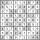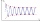# The sum

The sum of five consecutive odd numbers is 75. Find out the sum of the second and fourth of them.

s =  30

### Step-by-step explanation:Did you find an error or inaccuracy? Feel free to write us. Thank you!Tips to related online calculators
Do you have a linear equation or system of equations and looking for its solution? Or do you have a quadratic equation?

## Related math problems and questions:

• Even / odd numbersa / Using variable n write two consecutive odd numbers. b / the sum of three consecutive odd numbers is 333. What are this numbers?
• Sum of four numbersThe sum of four consecutive natural numbers is 114. Find them.
• Sum of sevenThe sum of seven consecutive odd natural numbers is 119. Determine the smallest of them.
• Even/odd numbersWhat is the product of 0.97 and the next odd decimal number?
• Odd numbersThe sum of four consecutive odd numbers is 1048. Find those numbers ...
• Digit sumFind the smallest natural number n, the digit sum of which is equal to 37.
• Have solutionThe sum of four consecutive even numbers is 92. Determine these numbers.
• Four multiplesFour multiples of 6 he writes the following 12  24  56  72, which is correct?
• The quotientThe quotient of g and 55 is the same as 279. What is g?
• Dropped sheetsThree consecutive sheets dropped from the book. The sum of the numbers on the pages of the dropped sheets is 273. What number has the last page of the dropped sheets?
• Last pageTwo consecutive sheets dropped out of the book. The sum of the numbers on the sides of the dropped sheets is 154. What is the number of the last page of the dropped sheets?
• The largest divisorThe largest number which divides 60 and 75 leaving remainders 8 and 10 respectively is:
• Sum 1-6Find the sum of the geometric progression 3, 15, 75,… to six terms.
• The sum 12The sum of 3 mixed numbers is 20 13/15. two of the numbers are 6 1/3 and 7 5/6. what is the third number?
• Common divisorsFind all common divisors of numbers 30 and 45.
• Unknown number 5I think of an unknown number. If we enlarge it five times, then subtract 3, and the result decreases by 75%, we get one greater than the number. What number am I thinking of?
• GroupsIn the 6th class there are 60 girls and 72 boys. We want to divide them into groups so that the number of girls and boys is the same. How many groups can you create? How many girls will be in the group?